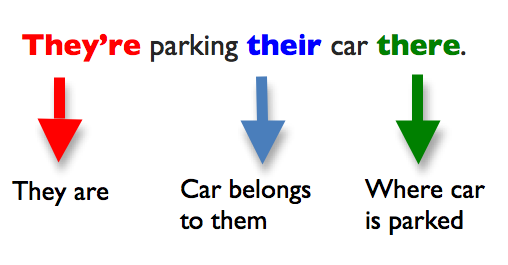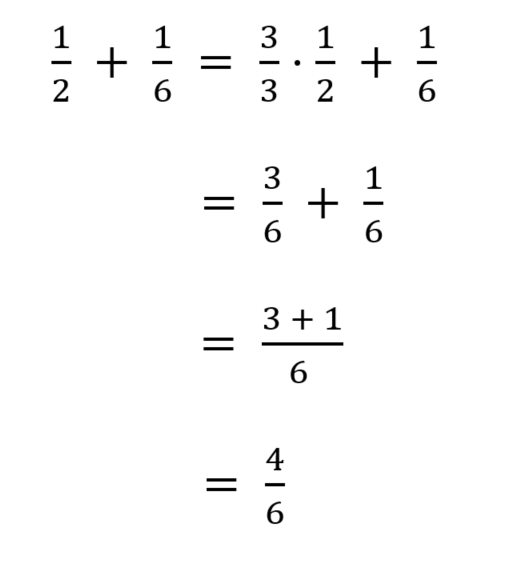# HESI A2 Tips — hesi a2 math

## The Most-Tested Topics of the HESI A2 nursing entrance examHow do I study for the HESI? There are several different topics the HESI nursing entrance exam will cover. What’s important to remember is that it is more of a foundational exam than minute details. We have broken down each section into general concepts. These topics are almost ALWAYS asked on the HESI Exam. We recommend you look up each vocabulary word or concept and look up the chapter summary of each theory. How Do I Know Which Subjects To Study For The HESI? For example, if you see Body Planes, look up the body planes chapter of your anatomy textbook...

Read more →

## How To Solve Ratios in the HESI A2 Math Section

hesi a2 mathThose are some delicious looking jelly beans! What do you think is the ratio of red jelly beans to blue jelly beans? What about pink jelly beans to whole bowls? Knowing how to solve for ratios is critical when taking the HESI A2 Math section. And we'll show you how to do it: Solving for Ratios  A ratio is a relationship between two numbers indicating how many times the first number contains the second.  For example, if the ratio of men to women is 1  to 5 then we know that for each man, there are 5 women.  For example,...

Read more →

## Solving Word Fraction Problems

hesi a2 mathFractions can get pretty hairy if you are unsure on how to break down a word problem. The HESI A2 math section is FILLED with fractions. It's kind of like that quote from Forest Gump, except it's more like: adding fractions, subtracting fractions, dividing fractions, multiplying fraction, percentages and fractions, rations and fractions, etc. Craig has 1/2 cup of juice. Joe has 1/6 cup more of juice than Craig. How much juice does Joe have? Solution: Joe has 1/2 + 1/6 cup of juice. We need to calculate the sum. Notice that the denominators are different. Step 1: Find the...

Read more →